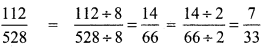Tamilnadu State Board New Syllabus Samacheer Kalvi 8th Maths Guide Pdf Chapter 1 Numbers Ex 1.1 Textbook Questions and Answers, Notes.

## Tamilnadu Samacheer Kalvi 8th Maths Solutions Chapter 1 Numbers Ex 1.1

Question 1.
Fill in the blanks:
(i) $$\frac{-19}{5}$$ lies between the integers _________ and _________ .
-4 and -3

(ii) The decimal form of the rational number $$\frac{15}{-4}$$ is _________ .
-3.75(iii) The rational numbers $$\frac{-8}{3}$$ and $$\frac{8}{3}$$ are equidistant from _________.
0

(iv) The next rational number in the sequence $$\frac{-15}{24}, \frac{20}{-32}, \frac{-25}{40}$$ is _________.
$$\frac{30}{-48}$$

(v) The standard form of $$\frac{58}{-78}$$ is _________.
$$\frac{-29}{39}$$

Question 2.
Say True or False
(i) 0 is the smallest rational number.
False

(ii) $$\frac{-4}{5}$$ lies to the left of $$\frac{-3}{4}$$.
True

(iii) $$\frac{-19}{5}$$ is greater than $$\frac{15}{-4}$$.
False

(iv) The average of two rational numbers lies between them.
True

(v) There are an unlimited number of rational numbers between 10 and 11.
TrueQuestion 3.
Find the rational numbers represented by each of the question marks marked on the following number lines.
(i)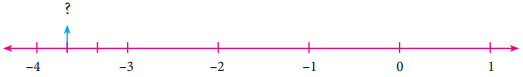The number lies between —3 and 4. The unit part between -3 and -4 is divided into 3 equal parts and the second part is asked.
∴ The required number is -3 $$\frac{2}{3}=-\frac{11}{3}$$

(ii)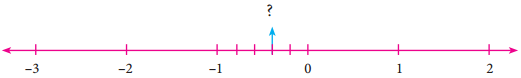The required number lies between 0 and -1. The unit part between 0 and -1 is divided
into 5 equal parts, and the second part is taken.
∴ The required number is $$-\frac{2}{5}$$

(iii)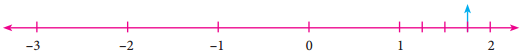The required number lies between 1 and 2. The unit part between 1 and 2 is divided into 4 equal parts and the third part is taken.
∴ The required number is 1$$\frac{3}{4}=\frac{7}{4}$$

Question 4.
The points S, Y, N, C, R, A, T, I and O on the number line are such that CN=NY=YS and RA=AT=TI=IO. Find the rational numbers represented by the letters Y, N, A, T and I.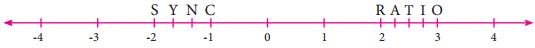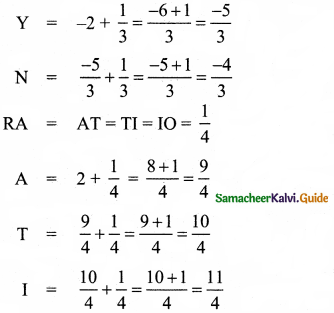Question 5.
Draw a number line and represent the following rational numbers on it.
(i) $$\frac{9}{4}$$
(ii) $$\frac{-8}{3}$$
(iii) $$\frac{-17}{-5}$$
(iv) $$\frac{15}{-4}$$
(i) $$\frac{9}{4}$$
$$\frac{9}{4}=2 \frac{1}{4}$$
∴ $$\frac{9}{4}$$ lies between 2 and 3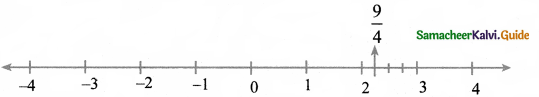(ii) $$\frac{-8}{3}$$
$$\frac{-8}{3}=-2 \frac{2}{3}$$
$$-2 \frac{2}{3}$$ lies between -2 and 3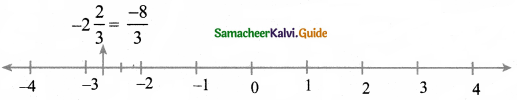(iii) $$\frac{-17}{-5}$$
$$\frac{-17}{-5}=3 \frac{2}{5}$$
$$3 \frac{2}{5}$$ lies between 3 and 4 in the number line.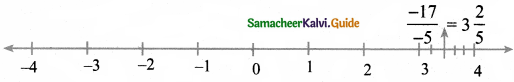(iv) $$\frac{15}{-4}$$
$$\frac{15}{-4}=-3 \frac{3}{4}$$
$$-3 \frac{3}{4}$$ lies between -3 and -4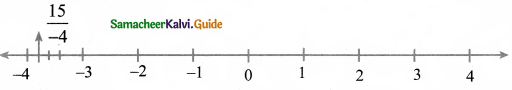Question 6.
Write the decimal form of the following rational numbers.
(i) $$\frac{1}{11}$$
(ii) $$\frac{13}{4}$$
(iii) $$\frac{-18}{7}$$
(iv) $$1 \frac{2}{5}$$
(v) $$-3 \frac{1}{2}$$
(i) $$\frac{1}{11}$$
$$\frac{1}{11}$$ = 0.0909….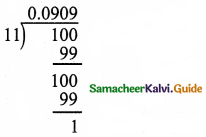(ii) $$\frac{13}{4}$$
$$\frac{13}{4}$$ = 3.25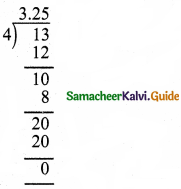(iii) $$\frac{-18}{7}$$
$$\frac{-18}{7}$$ = -2.571428571428….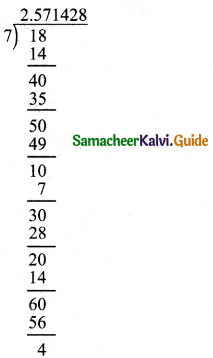(iv) $$1 \frac{2}{5}$$
$$1 \frac{2}{5}=\frac{7}{5}$$ = 1.4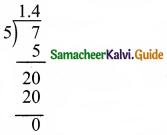(v) $$-3 \frac{1}{2}$$
$$-3 \frac{1}{2}=-\frac{7}{2}=-3.5$$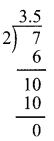Question 7.
List any five rational numbers between the given rational numbers.
(i) 2 and 0
(ii) $$\frac{-1}{2}$$ and $$\frac{3}{5}$$
(iii) $$\frac{1}{4}$$ and $$\frac{7}{20}$$
(iv) $$\frac{-6}{4}$$ and $$\frac{-23}{10}$$
(i) 2 and 0
i.e., $$\frac{-2}{1}$$ and $$\frac{0}{1}$$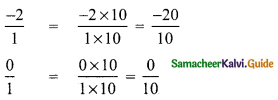∴ Five rational number between $$\frac { -20 }{ 10 }$$ (= -2) and $$\frac { 0 }{ 10 }$$ (= 0) are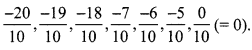(ii) $$\frac{-1}{2}$$ and $$\frac{3}{5}$$
LCM of 2 and 5 = 2 × 5 = 10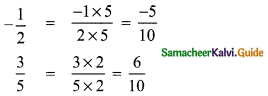∴ Five rational number between(iii) $$\frac{1}{4}$$ and $$\frac{7}{20}$$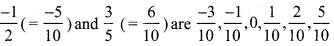∴ Five rational number between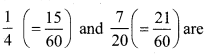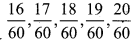(iv) $$\frac{-6}{4}$$ and $$\frac{-23}{10}$$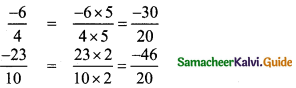∴ Five rational number between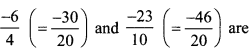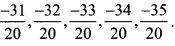Question 8.
Use the method of averages to write 2 rational numbers between $$\frac{14}{5}$$ and $$\frac{16}{3}$$
The average of a and b is $$\frac { 1 }{ 2 }$$(a + b)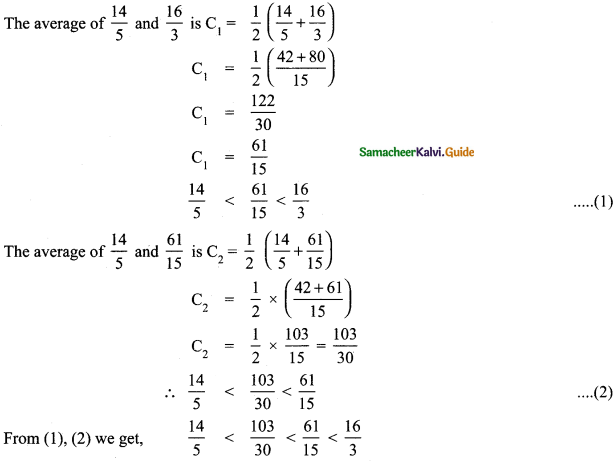Question 9.
Compare the following pairs of rational numbers.
(i) $$\frac{-11}{5}, \frac{-21}{8}$$
(ii) $$\frac{3}{-4}, \frac{-1}{2}$$
(iii) $$\frac{2}{3}, \frac{4}{5}$$
(i) $$\frac{-11}{5}, \frac{-21}{8}$$
LCM of 5, 8 is 40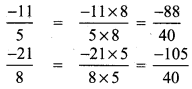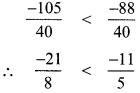(ii) $$\frac{3}{-4}, \frac{-1}{2}$$
LCM of 4 and 2 = 4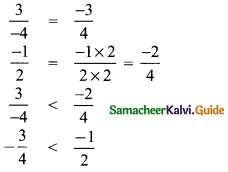(iii) $$\frac{2}{3}, \frac{4}{5}$$
LCM of 3 and 5 is 15.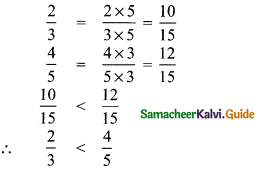Question 10.
Arrange the following rational numbers in ascending and descending order.
(i) $$\frac{-5}{12}, \frac{-11}{8}, \frac{-15}{24}, \frac{-7}{-9}, \frac{12}{36}$$
(ii) $$\frac{-17}{10}, \frac{-7}{5}, 0, \frac{-2}{4}, \frac{-19}{20}$$
(i) $$\frac{-5}{12}, \frac{-11}{8}, \frac{-15}{24}, \frac{-7}{-9}, \frac{12}{36}$$
LCM of 12, 8, 24, 9, 36 is 4 × 3 × 2 × 3 = 72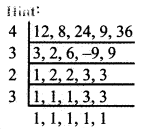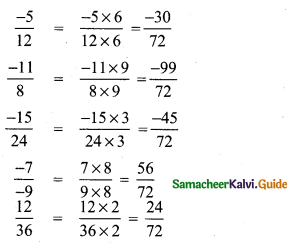Now comparing the numerators – 30, – 99, -45, 56, 24 we get 56 > 24 > – 30 > – 45 > – 99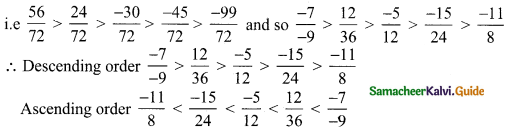(ii) $$\frac{-17}{10}, \frac{-7}{5}, 0, \frac{-2}{4}, \frac{-19}{20}$$
LCM of 10, 5, 4, 20 is 5 × 2 × 2 = 20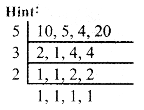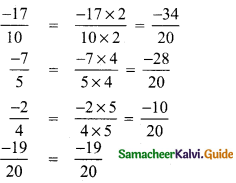Negative numbers are less than zero.
∴ Arranging the numerators we get
– 34 < – 28 < – 19 < – 10 < 0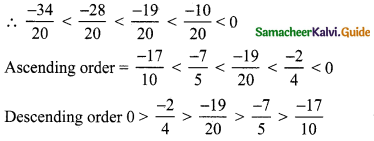Objective Type Questions:

Question 11.
The number which is subtracted from $$\frac{-6}{11}$$ to get $$\frac{8}{9}$$ is _________ .
(A) $$\frac{34}{99}$$
(B) $$\frac{-142}{99}$$
(C) $$\frac{142}{99}$$
(D) $$\frac{-34}{99}$$
(B) $$\frac{-142}{99}$$
Hint:
Let x be the number to be subtracted
$$\frac{-6}{11}-x$$ = $$\frac{8}{9}$$
$$\frac{-6}{11}-\frac{8}{9}$$ = x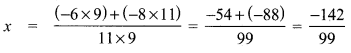Question 12.
Which of the following pairs is equivalent?
(A) $$\frac{-20}{12}, \frac{5}{3}$$
(B) $$\frac{16}{-30}, \frac{-8}{15}$$
(C) $$\frac{-18}{36}, \frac{-20}{44}$$
(D) $$\frac{7}{-5}, \frac{-5}{7}$$
(B) $$\frac{16}{-30}, \frac{-8}{15}$$
Hint: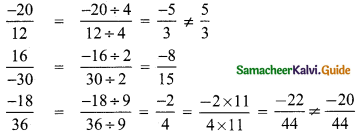∴ $$\frac{16}{-30}$$ and $$\frac{-8}{15}$$Question 13.
$$\frac{-5}{4}$$ is a rational number which lies between _________ .
(A) 0 and $$\frac{-5}{4}$$
(B) -1 and 0
(C) -1 and -2
(D) -4 and -5
(C) -1 and -2
Hint:
$$\frac{-5}{4}$$ = -1 $$\frac{1}{4}$$
∴ $$\frac{-5}{4}$$ lies between -1 and -2.

Question 14.
Which of the following rational numbers is the greatest?
(A) $$\frac{-17}{24}$$
(B) $$\frac{-13}{16}$$
(C) $$\frac{7}{-8}$$
(D) $$\frac{-31}{32}$$
(A) $$\frac{-17}{24}$$
Hint:
LCM of 24, 16, 8, 32 = 8 × 2 × 3 × 2 = 96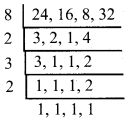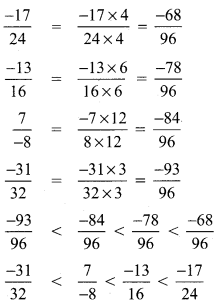∴ $$\frac{-17}{24}$$ is the greatest numberQuestion 15.
The sum of the digits of the denominator in the simplest form of is $$\frac{112}{528}$$ is _________ .
(A) 4
(B) 5
(C) 6
(D )7